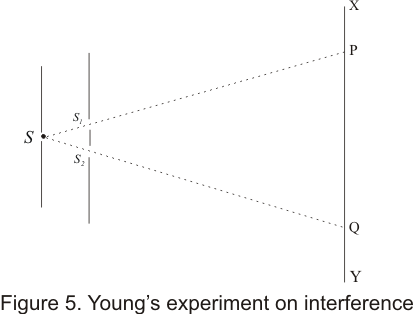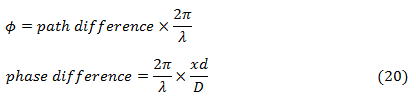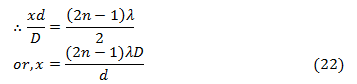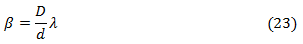# Huyghen's principle and interference of light

## 9)Young Double slit experiment

• Young in 1801 demonstrated interference phenomenon through double slit experiment
• In his experiment ,he divided a single wave front into two and these two slit wave fronts acts as if they emerged from two sources having fixed relationship
• when these two waves were allowed to interfere ,they produce a sustained interference pattern
• In his original experiment he illuminates a pin hole S using a light source and light diverging from pinhole which contains two sets of pinholes S1 and S2 equidistant from S and very close to one another as shown below in the figure• Two two sets of spherical waves coming out of the pin holes S1 and S2 were coherent and interfered with each other to form a symmetrical pattern of varying intensity on screen XY
• This interference pattern disappear when any one of the pinholes S1 or S2 is closed
• Young used the superposition principle to explain the interference pattern and by measuring the distance between the fringes he managed to calculate the wavelength of light.

## 10) Theory of interference fringes

• In young's double slit experiment ,light wave produce interference pattern of alternate bright and dark fringes or interference band
• To find the position of fringes, their spacing and intensity at any point P on screen XY .Consider the figure given below• Here S1 or S2 two pin holes of YDS interference experiment and position of maxima and minima can be determined on line XOY parallel to Y-axis and lying on the plane parallel to S,S1 or S2
• Consider a point P on XY plane such that CP = x.The nature of interference between two waves reaching point P depends on the path difference S2P-S1P
• from figure (6)for x, d<<< D , S1P+S2P =2D
with negligible error included , path difference would beAnd corresponding phase difference between wave isi) Condition of bright fringes(constructive interference)
• If the path difference (S2P-S1P) is even multiple of λ/2,the point P is bright• Equation (21) gives the condition for bright fringes or constructive interference
ii) Condition for dark fringes (destructive interference)
• If the path difference is an odd multiple of λ/2,the Point P is dark. So,• Equation (22) gives the condition for dark fringes or destructive interference
• From equations (21) and (22) ,we can get position of alternate bright and dark fringes respectively
• Distance between two consecutive bright fringes is given by• Thus the distance between two successive dark and bright fringes is same. This distance is known as fringe width and is denoted by β. Thus